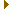# mightymandel v16

## GPU-based Mandelbrot set explorer

complex.h File Reference

### (v16)

Helpers for complex number arithemetic with mpfr. More...

#include <mpfr.h>Include dependency graph for complex.h:This graph shows which files directly or indirectly include this file:

Go to the source code of this file.

## Data Structures

struct  C
Complex number type. More...

## Macros

#define c_set_prec(o, p)
Set the precision of both parts of a complex number.
#define c_set(o, l)
Set both parts of a complex number.
#define c_mag2(o, l, t1, t2)
Compute complex magnitude squared.
#define c_sqr(o, l, t1, t2, t3)
Square a complex number.
#define c_mul(o, l, r, t1, t2, t3, t4)
Multiply two complex numbers.
#define c_mul_2ui(o, l, r)
Multiply a complex number by a power of two.

## Typedefs

typedef mpfr_t R
Real number type.

## Detailed Description

Helpers for complex number arithemetic with mpfr.

All variables must already be mpfr_init2()d with appropriate precision. Implemented as CPP macros as a last resort (the C++ version used reference parameters).

Definition in file complex.h.

## Data Structure Documentation

 struct C

Complex number type.

Definition at line 27 of file complex.h.

Data Fields
R x Real part.
R y Imaginary part.

## Macro Definition Documentation

 #define c_add ( o, l, r )
Value:
do{\
}while(0)

Parameters
 o The complex number for output. l The first complex number input. r The second complex number input.

Definition at line 75 of file complex.h.

Referenced by fpxx_approx_do().

 #define c_mag2 ( o, l, t1, t2 )
Value:
do{\
mpfr_sqr(t1, l.x, MPFR_RNDN);\
mpfr_sqr(t2, l.y, MPFR_RNDN);\
}while(0)

Compute complex magnitude squared.

Parameters
 o The real number for output. l The complex number input. t1 A real temporary variable. t2 A real temporary variable.

Definition at line 62 of file complex.h.

Referenced by fpxx_approx_do().

 #define c_mul ( o, l, r, t1, t2, t3, t4 )
Value:
do{\
mpfr_mul(t1, l.x, r.x, MPFR_RNDN);\
mpfr_mul(t2, l.y, r.y, MPFR_RNDN);\
mpfr_mul(t3, l.x, r.y, MPFR_RNDN);\
mpfr_mul(t4, l.y, r.x, MPFR_RNDN);\
mpfr_sub(o.x, t1, t2, MPFR_RNDN);\
}while(0)

Multiply two complex numbers.

Parameters
 o The complex number for output. l The first complex number input. r The first complex number input. t1 A real temporary variable. t2 A real temporary variable. t3 A real temporary variable. t4 A real temporary variable.

Definition at line 108 of file complex.h.

Referenced by fpxx_approx_do().

 #define c_mul_2ui ( o, l, r )
Value:
do{\
mpfr_mul_2ui(o.x, l.x, r, MPFR_RNDN);\
mpfr_mul_2ui(o.y, l.y, r, MPFR_RNDN);\
}while(0)

Multiply a complex number by a power of two.

Parameters
 o The complex number for output. l The complex number input. r The unsigned integer power of two.

Definition at line 124 of file complex.h.

Referenced by fpxx_approx_do().

 #define c_set ( o, l )
Value:
do{\
mpfr_set(o.x, l.x, MPFR_RNDN);\
mpfr_set(o.y, l.y, MPFR_RNDN);\
}while(0)

Set both parts of a complex number.

Parameters
 o The complex number to modify. l The complex number to use as source.

Definition at line 49 of file complex.h.

Referenced by fpxx_approx_do().

 #define c_set_prec ( o, p )
Value:
do{\
mpfr_set_prec(o.x, p);\
mpfr_set_prec(o.y, p);\
}while(0)

Set the precision of both parts of a complex number.

Parameters
 o The complex number. p The precision.

Definition at line 38 of file complex.h.

Referenced by fpxx_approx_do().

 #define c_sqr ( o, l, t1, t2, t3 )
Value:
do{\
mpfr_sqr(t1, l.x, MPFR_RNDN);\
mpfr_sqr(t2, l.y, MPFR_RNDN);\
mpfr_mul(t3, l.x, l.y, MPFR_RNDN);\
mpfr_sub(o.x, t1, t2, MPFR_RNDN);\
mpfr_mul_2ui(o.y, t3, 1, MPFR_RNDN);\
}while(0)

Square a complex number.

Parameters
 o The complex number for output. l The complex number input. t1 A real temporary variable. t2 A real temporary variable. t3 A real temporary variable.

Definition at line 89 of file complex.h.

Referenced by fpxx_approx_do().

## Typedef Documentation

 typedef mpfr_t R

Real number type.

Definition at line 22 of file complex.h.